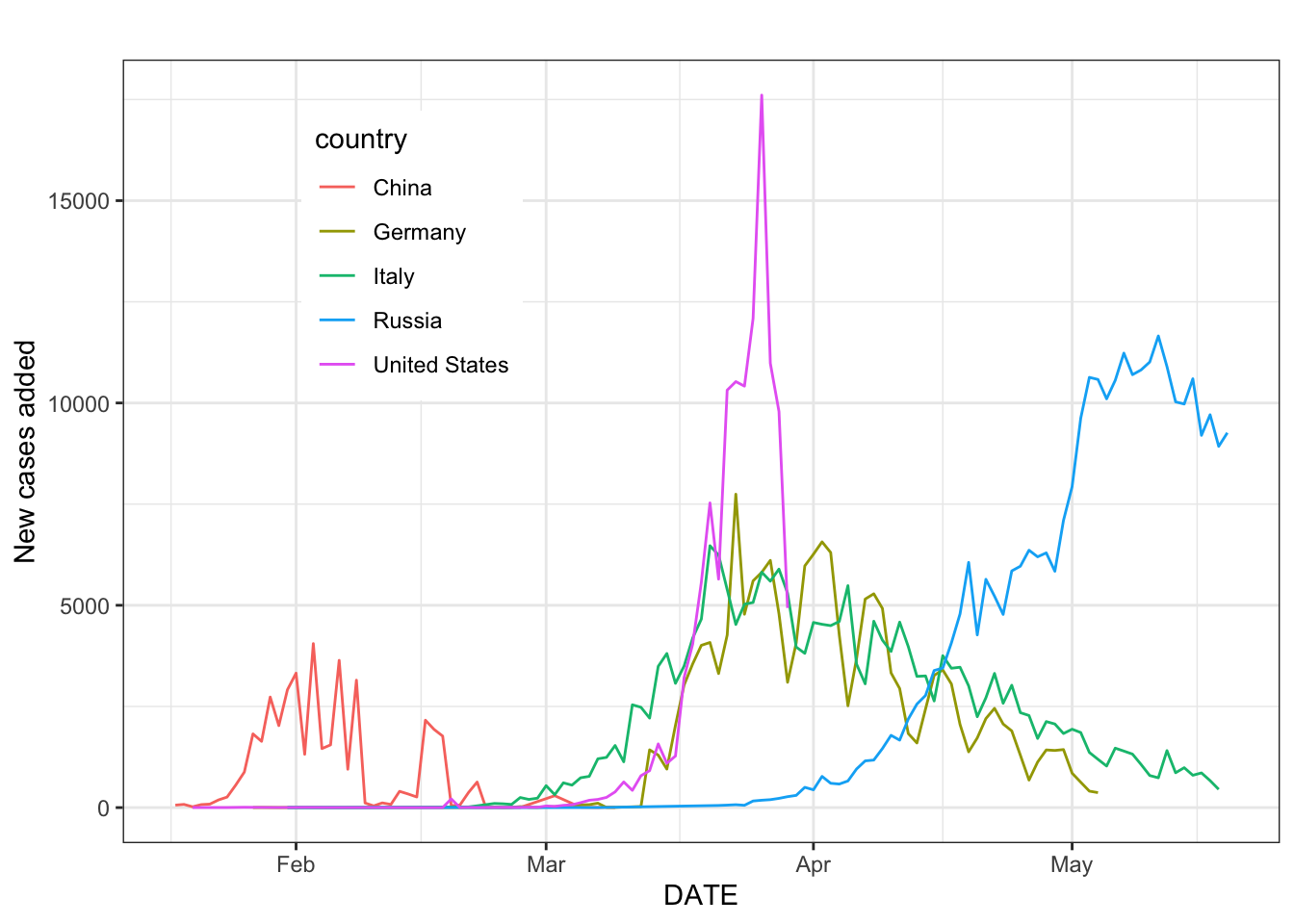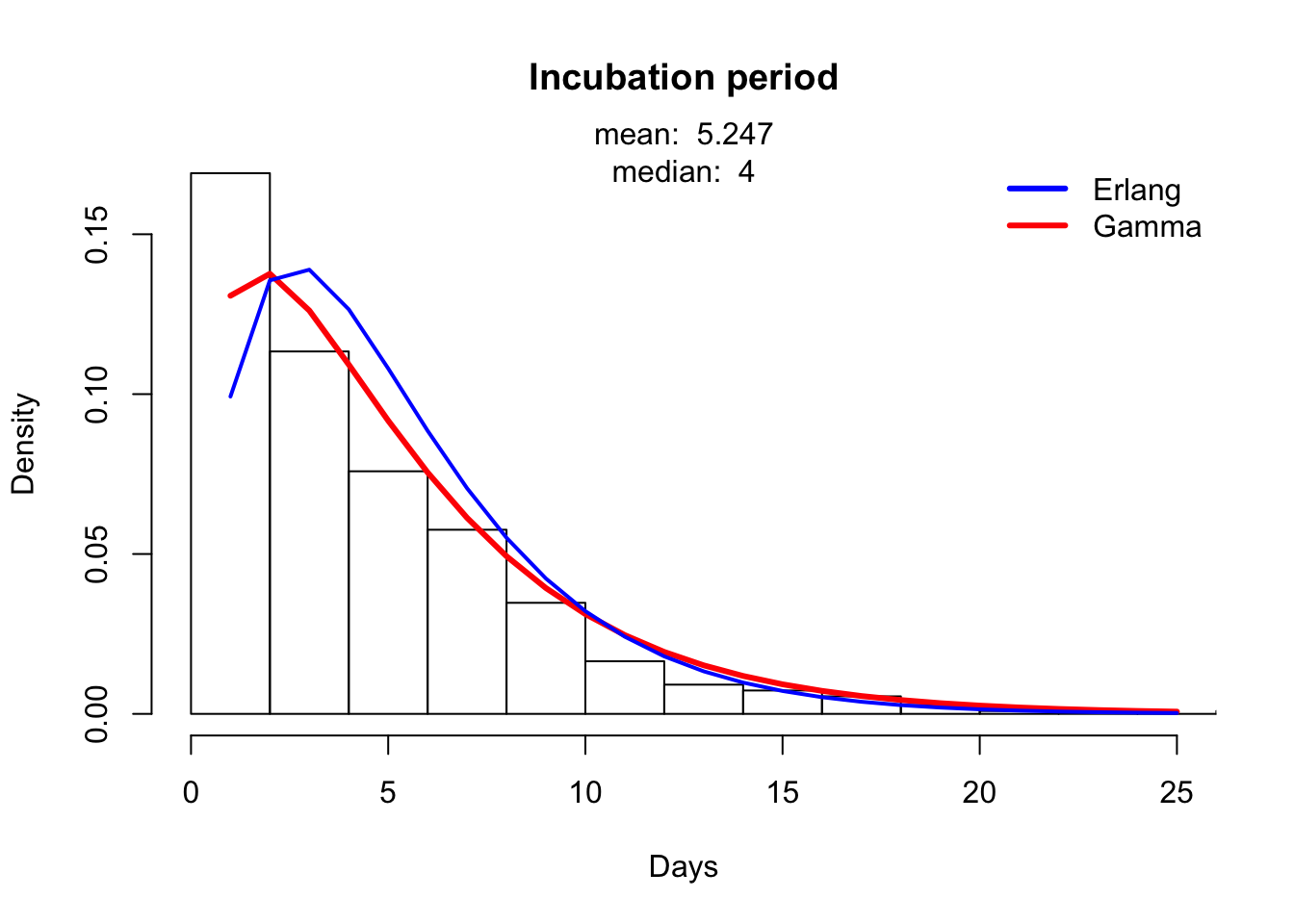## Parameters

### Summary

COVID19 is an emerging pathogen and much of its epidemiology remains poorly understood. Identifying the key processes that shape transmission and estimating the relevant model parameters is therefore an important task. This document presents arguments and analysis to support the estimation of a number of key quantities:

• Incubation period ($$1/\sigma$$)
• Recovery/isolation rate ($$1/\gamma$$)
• Interval from hospitalization to notification ($$\tau_1$$) and notification rate ($$\eta$$)
• Case fatality rate ($$m$$)
• Basic reproduction number ($$R_0$$)
• Case detection rate ($$q$$)
• Transmissibility ($$\beta$$)

To estimate these parameters, we use the following resources:

1. A record of case reports at the province level in China maintained by the CEID at the University of Georgia
2. A global linelist maintained by Be Outbreak Prepared (BOP) This dataset was preceded by the “Moritz Kraemer” linelist and has since been published in the Lancet

The Be Outbreak Prepared Linelist contains individual-level information on COVID19 cases. The BOP dataset is not comprehensive, there are currently ~1.2 million cases in it, but many cases have limited information and many cases are likely not represented in the dataset. Russia, Italy, Germay, the US, France, Belgium, and China have the most cases in the linelist. New linelist cases in the US cut off at the beginning of April. The number of new cases over time in 5 countries is shown below.Findings are preliminary and subject to change, pending changes in the underlying data. Results have not been peer-reviewed, but have been prepared to a professional standard with the intention of providing useful information about a rapidly developing event.

### Incubation period

Work on distribution of the incubation period (time from exposure to symptom onset) has estimated it to vary from around 4-12 days (MIDAS network parameter list). Some peer-reviewed estimates of the incubation period include:

We use the Be Prepared linelist to estimate incubation period. From this dataset, we assume incubation period was the delay from day of travel from Wuhan and the symptom onset. Only cases outside Wuhan were used in this calculation since we used travel to Wuhan as the time of exposure. We fit gamma and Erlang distributions to these intervals. The Erlang distribution is a special case of a gamma distribution with an integer shape parameter. The PDF of the Erlang distribution is

$\begin{equation} f(x; k, \lambda) = \frac{\lambda^{k}x^{k-1}e^{-\lambda x}}{(k-1)!} \end{equation}$

for rate parameter $$\lambda \geq 0$$. We estimate the Erlang distribution of isolation intervals numerically and analytically by optimizing the negative log likelihood. The MLE of this distribution can be solved by finding the derivative of the log likelihood function:

$\begin{equation} L = n k log(\lambda) + (k-1) \sum_{i=1}^{n}{x_i} - \lambda \sum_{i=1}^{n}{x_i} - n log(k-1)! \end{equation}$

which has solution $$\hat{\lambda}=\frac{k}{\sum_{i=1}^{n}{x_i}}$$.

The below histograms show incubation period from the linelist outside of Wuhan, with periods longer than 0 and shorter than 50 days. We filtered the linelist to include only cases that had known exposures in: Wuhan, high risk country, contact with a confirmed case. Hubei, Hubei province, Italy, and USA.

Cases used to estimate incubation period by country.
Country Cases
China 230
Mexico 228
Japan 18
Cuba 16
South Korea 12
Australia 10
United States 6
Brazil 6
South Africa 2
Philippines 2
Cambodia 2
Spain 1
France 1
Chile 1
Cases used to estimate incubation period by age and sex.
age_class female male
0-15 2 4 NA
15-30 44 56 NA
30-45 68 129 3
45-60 55 96 NA
60+ 42 43 1
NA 2 2 NAIncubation period for US cases in the Be Outbreak Prepared linelist.